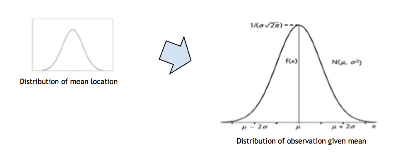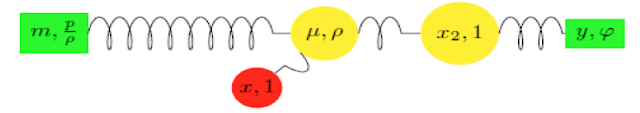## Thursday, October 31, 2013

### Hooke's Law and the Kalman filter. A little "spring theory" emphasizing the connection between statistics and physics.

Readers will be familiar with statistical mechanics. But what about mechanical statistics?

This post is purely expository and concerns the simplest one dimensional Kalman filter. You'll recall the setup. We periodically observe Brownian motion subject to gaussian measurement error and attempt to infer the ground truth from those measurements. But rather than dive into a bunch of conditional expectations for gaussian linear systems we'll look for some physical intuition. We'll pretend that we've been robbed of our computers and are forced to create analog varieties just as in the good old days.

We make an observation that isn't always stressed up front in the statistical literature (Harrison and West for example) or control systems perspective (such as you will find at the at wikipedia  entry for "Kalman filter", for example). Then we pursue the analogy between statistics and physics a little further, and show how the updating of a location estimate of a gaussian distribution amounts to a combination of center of mass and reduced mass calculations.

The perspective we arrive at is that most statistical calculations of a filtering variety are directly mapped to a corresponding physical system containing both fixed and free masses. Resolving these physical systems requires a change of reference frame. The calculations are therefore, somewhat unsurprisingly, precisely "center of mass" and "reduced mass" calculations from physics.

Kalman filter equations are just a center of mass calculation

Suppose the prior estimate of location for a particle is $$m$$ and the prior covariance is $$P$$. Suppose we make an observation $$y$$ with error variance $$R$$. Our posterior belief is gaussian with location $$m'$$ say and variance $$P'$$. The update is usually written \begin{eqnarray} m' & = & m + K ( y - m ) \\ P' & = & P(1-K), \ \ {\rm where} \\ K & = & \frac{P}{P+R} \end{eqnarray} However it is in many ways more natural to use the inverses of covariances instead. If we write $$\varphi = 1/R$$, $$p = 1/P$$ and $$p' = 1/P'$$ and multiply by through by $$\frac{P+R}{PR}$$ we notice that the Kalman filter update is merely a center of mass calculation: \begin{eqnarray} m' & = & \frac{m/P + y/R} { 1/R + 1/P } = \frac{ pm + \varphi y }{ \varphi + p } \\ p' & = & \frac{1}{P'} = \frac{P+ R}{PR} = \frac{1}{P} + \frac{1}{R} = \varphi + p \end{eqnarray} The analogy works if we treat precision as mass. And in what follows we'll be equally interested in the analogy between force and the derivative of the negative log likelihood function.

This table suggests that in a gaussian world force is linear in distance. And it true that we can construct an analogue Kalman smoother with rods and springs as follows:An "analogue" gaussian smoother using perfect Hookean springs
Minimizing energy corresponds to minimizing maximizing log-likelihood. And setting the derivative of log-likelihood to zero corresponds to finding the equilibrium where forces cancel out.

Futhermore the fact that combining two pieces of evidence for one latent variable can sometimes be as simple as merging the two observations at their "center of precision" corresponds to a nice accident when forces grow linearly with distance: the impact of two masses on a third is unchanged if they coalesce at their center of mass.

But there is more to the story...

Reading a "spring diagram" in a Hookean universe

To demonstrate a richer physical analogy we consider next a Gaussian distribution whose location is assumed unknown, but also gaussian.Figure 1. Hierarchical model where location of a gaussian distribution is itself gaussian

Suppose our prior is \begin{eqnarray} P( x | \mu ) & \propto & e^{-\rho(x-\mu)^2} \\ P(\mu) & \propto & e^{-p(\mu-m)^2} \end{eqnarray} where this time $$m$$ represents our guess as to the location of the center of the distribution. Symbolically we might represent the prior with the following diagram.Figure 2. Spring diagram representing prior knowledge of the location of a gaussian distribution

Here the tuples represent location and precision, or if you prefer, position and mass. It isn't an analogy. If we assume attractive forces vary as a Hookean law, which is to say proportional to the product of masses and distance: \begin{equation} Force \propto M_1 M_2 d \end{equation} then in the example above the yellow and green masses witness attractive force and potential energy given by \begin{eqnarray*} {\rm Force} & = & \frac{p}{\rho} \rho |m - \mu| = p |m - \mu |,\ \ {\rm and\ thus} \\ {\rm Energy} & = & \frac{1}{2} p (m - \mu )^2\\ \end{eqnarray*} Since energy is the negative log likelihood, we "read" the diagram as $$P( x | \mu ) \propto e^{-\rho(x-\mu)^2}$$. Similarly the spring between the yellow mass and red "test particle" is read $$P(\mu) \propto e^{-p(\mu-m)^2}$$. The system therefore represents the hierarchical model and indeed, it is an exact physical analogue. So in what follows we will ask the following question: what force does the test particle feel as we add other masses to the picture?

Simplifying  a spring diagram using reduced mass

The game begin in earnest when we introduce noisy evidence of our unknown location parameter $$\mu$$ for our mysterious distribution. Suppose we take a draw from said distribution $$x_2$$. Suppose we don't observe $$x_2$$ itself but instead, a noisy measurement $$y$$ whose precision (or "mass", if you will) is $$\varphi$$. The noisy measurement's distribution conditional on $$x_2$$ is $$P(y|x_2) \propto e^{-\varphi(y-x_2)^2}$$ and corresponds to the following spring diagram.Figure 3. Spring diagram representing noisy evidence
As suggested in the diagram, we will attach the evidence to the latent variable $$\mu$$ as follows:Figure 4. Prior location belief plus a noisy measurement
Our task is simplification of this system until, from the perspective of the test mass, it resembles the form of the prior we began with. We should think of the observed evidence (green rectangles) as fixed points whereas the yellow circles represent unknown quantities that are free to move.

We ought to recall here the rules for combining springs in series, or to be more direct, the "reduced mass" trick for replacing a three body problem with a two body problem. In either situation physics reminds us that the combined action of the rightmost two masses can be simplified:Figure 5. Prior belief plus a noisy measurement simplified using reduced mass

We replace the mass $$\phi$$ with a reduced mass $$\frac{\phi}{\phi+\rho}$$ because the intermediating unit mass reduces the pull. Since it is well covered elsewhere I will not derive the reduced mass expression but notice why the reduced mass makes sense in the limits. If $$\phi \rightarrow 0$$ the relative size of the yellow unit mass is huge and so the mass at $$\mu$$ hardly feels the pull from the green mass at $$y$$ at all. In the other extreme case, when $$\phi \rightarrow \infty$$, the unit mass is sucked into the green mass and is, for all intents and purposes, stationary. Thus it acts like a fixed unit mass pulling the mass at $$\mu$$ rather than a floating one.

We proceed to the final simplification of the diagram. This is pretty easy as the two green masses are inertial. Their impact on the yellow mass is equivalent to a single inertial mass at their center of mass. Thus:Figure 6. Simplification of Figure 4 by reduced mass and center of mass calculation.
Apologies here because the diagram contains a small error. The denominators should read $$\varphi + \rho$$, not $$\varphi + p$$. Evidently this takes the form of the prior system in Figure 2 and can be easily read. It states that our posterior belief about the location parameter $$\mu$$ is gaussian with mean $$m'$$ say and precision $$p'$$ say, where \begin{eqnarray*} m' & = & \frac{ m \frac{p}{\rho} + y \frac{\varphi}{\varphi+\rho} } { \frac{p}{\rho} + \frac{\varphi}{\varphi + \rho} } \\ p' & = & \frac{p}{\rho} + \frac{\varphi}{\varphi + \rho} \end{eqnarray*}
This closes the loop and demonstrates how updating can be performed for the hierarchical model in Figure 1.

Recovering the Kalman filter update

As a parting note, we see that the limit $$\rho \rightarrow \infty$$ leads to update equations \begin{eqnarray} p' & = & \frac{p}{\rho} + \frac{\varphi}{\varphi + \rho} = \frac{p+p/\rho + \varphi}{\varphi +\rho}  \rightarrow \varphi + p \\ m' & = & \frac{ m \frac{p}{\rho} + y \frac{\varphi}{\varphi+\rho} } { \frac{p}{\rho} + \frac{\varphi}{\varphi + \rho} }   \rightarrow \frac{ pm + \varphi y }{ \varphi + p } \end{eqnarray} which is the Kalman update as before. This is to be expected, since in the limit $$\rho \rightarrow \infty$$ the problem of locating a distribution with unknown location (noisily observed) shrinks down to the problem of locating a point mass with unknown location (noisily observed).